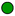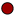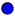Jr Math section of MathBitsNotebook.comFunctionsNGMS-6NGMS-7NGMS-8Extra
*
Function Basics (definition, vertical line test, calculator use, notation (opt))Represent & Compare Functions (descriptions, tables, equations, graphs, compare)Function Features (intercepts, increase/decrease, positive/negative, max/min)PRACTICE: • Basic Function Practice• Practice with Function Features• Practice with Comparing Functions• Practice with Linear and Non-Linear Functions• Function Word Problems Practice• Vocabulary - Word Guess Game (topical vocabulary game - iPad compatible)